# Test: Analog Electronics - 2

## 20 Questions MCQ Test GATE ECE (Electronics) 2023 Mock Test Series | Test: Analog Electronics - 2

Description
Attempt Test: Analog Electronics - 2 | 20 questions in 60 minutes | Mock test for Electronics and Communication Engineering (ECE) preparation | Free important questions MCQ to study GATE ECE (Electronics) 2023 Mock Test Series for Electronics and Communication Engineering (ECE) Exam | Download free PDF with solutions
QUESTION: 1

### Shown below is a timer 555 IC circuit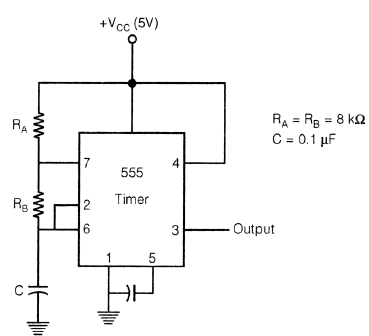Frequency of the waveform generated by the above circuit is

Solution: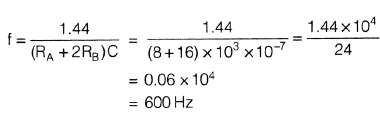QUESTION: 2

### The circuit shown in figure below represents: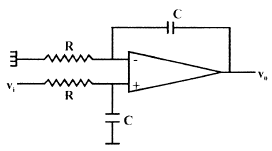Solution:

It is just by figure only. But one can also calculate transfer function.

*Answer can only contain numeric values
QUESTION: 3

### Consider the following feedback pair connection.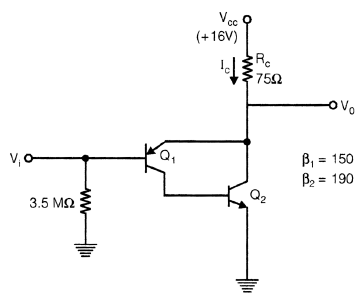Collector current IC is ____________ mA.

Solution: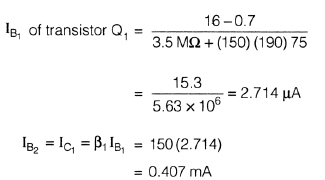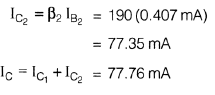QUESTION: 4

If for a given programmable OPAMP, G1 is the gain, when only S1 is closed and G2 is the gain when only S2 is closed, then relation between G1 & G2 is?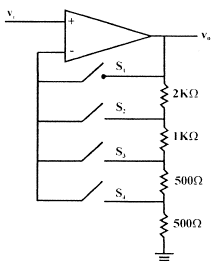Solution:

When S1 is closed G1 = 1 it behaves like a voltage follower circuit. When S2 s closed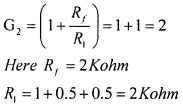QUESTION: 5

For given RC − Phase shift oscillator, by use of BJT if R1 = R2 = 10k, Rc = 2K, R = 2K, C = 0.01 µF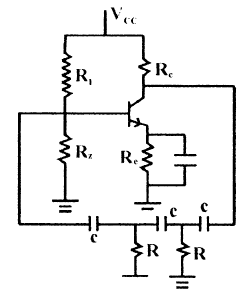Q.

What is Minimum value of hfe for possible oscillation?

Solution: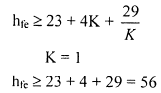QUESTION: 6

For given RC − Phase shift oscillator, by use of BJT if R1 = R2 = 10k, Rc = 2K, R = 2K, C = 0.01 µFQ.

What is frequency of oscillation for circuit?

Solution: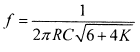QUESTION: 7

For a given CE, amplifier if β = 100, IC = 1 mA, RS = 1 KΩ, Rc = 5 KΩ, Re = 200 Ω, hie = 2.5 K.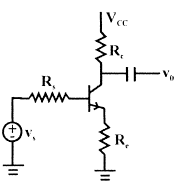Q.

Calculate value of voltage gain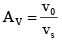Solution: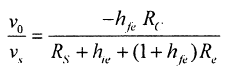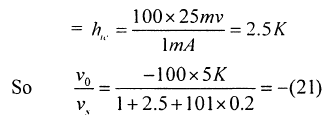QUESTION: 8

For a given CE, amplifier if β = 100, IC = 1 mA, RS = 1 KΩ, Rc = 5 KΩ, Re = 200 Ω, hie = 2.5 K.Q.

Calculate input impedance seen by source

Solution: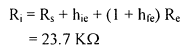QUESTION: 9

For the given FET, circuit if

RD = 20 kΩ rd = 50 kΩ
RS = 2 kΩ µ = 50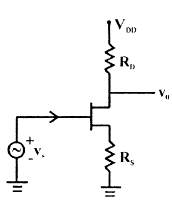Q.

Calculate value of v0/vs

Solution: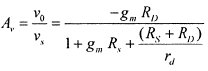QUESTION: 10

For the given FET, circuit if

RD = 20 kΩ rd = 50 kΩ
RS = 2 kΩ µ = 50Q.

Calculate value of output impedance

Solution:QUESTION: 11

The output voltage V0 for given circuit is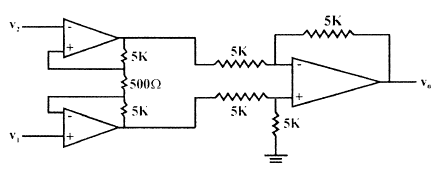Solution:*Answer can only contain numeric values
QUESTION: 12

A MOSFET amplifier circuit is as shown below:

If device parameter K = 15 µA/V2, then value of Transconductance of MOSFET is _________ ms.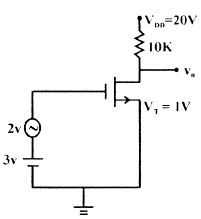Solution: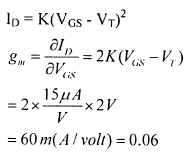QUESTION: 13

The 3-terminal linear voltage regulator is connected to a 10 ohm load resistor as shown in figure. If Vin is 10 volt, what is power dissipated in the Si transistor.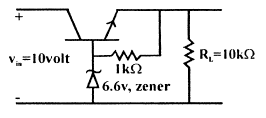Solution:
QUESTION: 14

A sinusoidal oscillator is built using an amplifier of real gain A, infinite input impedance and feedback (β) network is shown in figure. If Z = jX, then what is the value of z0.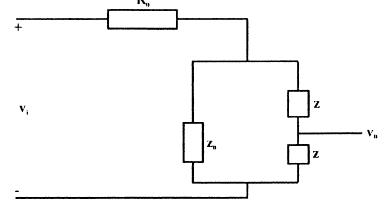Solution:

Z+Z+Z0 =0 ⇒ Z0 = - 2jX

QUESTION: 15

Shown below is a network, in collector feedback mode.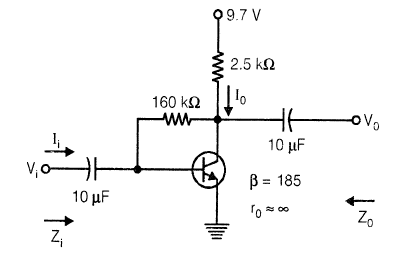Q.

The voltage gain Av is ……………., Assume re =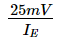Solution: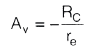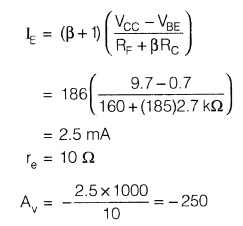QUESTION: 16

Shown below is a network, in collector feedback mode.Q.

The current gain Ai is ≈

Solution: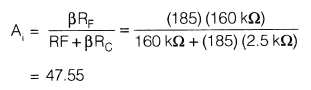QUESTION: 17

A power amplifier delivers 250 watt output at 50% efficiency. If ambient temp. is 25°C and maxm allowable junction temp. is 150°C, then what is value of Maximum thermal resistance which can be tolerated.

Solution: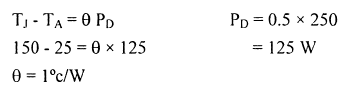*Answer can only contain numeric values
QUESTION: 18

Numerical Anser type question

Q.

For a BJT, CE-mode, Base to emitter capacitance (Cπ) is 10 times the collector to base capacitance (Cµ). If transistor is biased at quiescent collector current ICQ = 1 mA, and its short circuit unity gain frequency is 0.909 M rad/sec, then the value of Cπ is _______ nF.

Solution: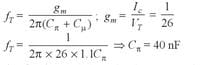QUESTION: 19

In voltage reference diodes:

Solution:

Voltage reference diode means zener diode

QUESTION: 20

What should be values of components R & R2 such that frequency of wein-bridge oscillator is 300 HZ, if C = 0.01 µF, R1 = 12 kΩ.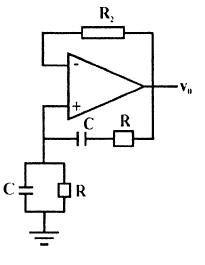Solution: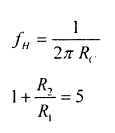Use Code STAYHOME200 and get INR 200 additional OFF Use Coupon Code